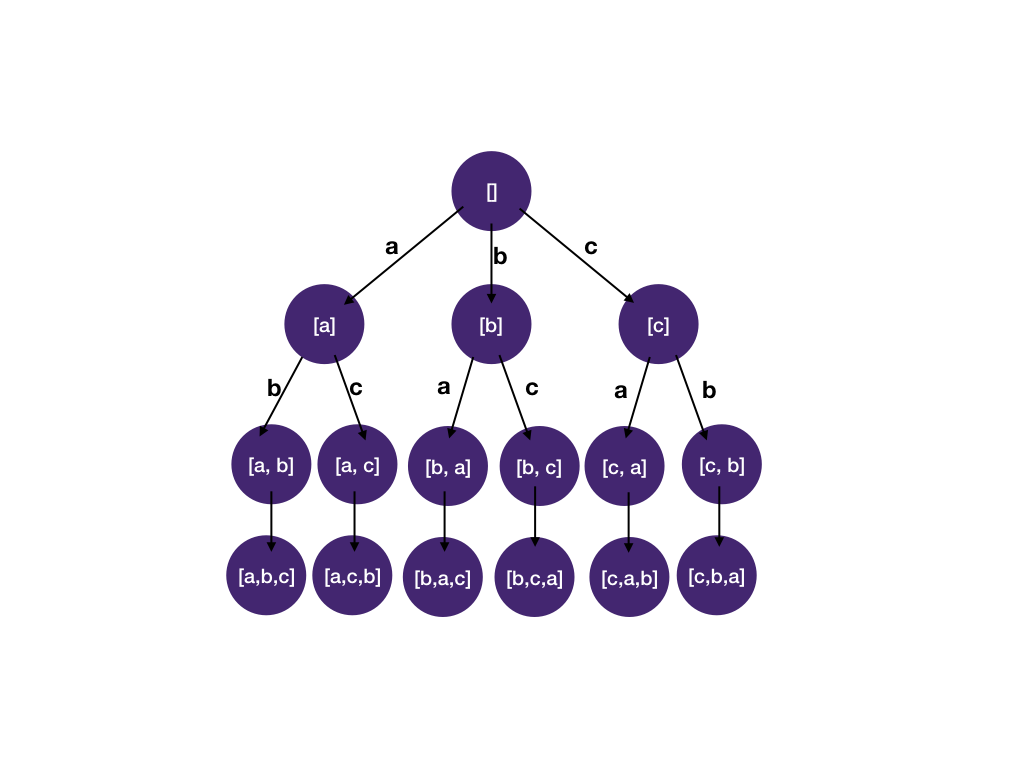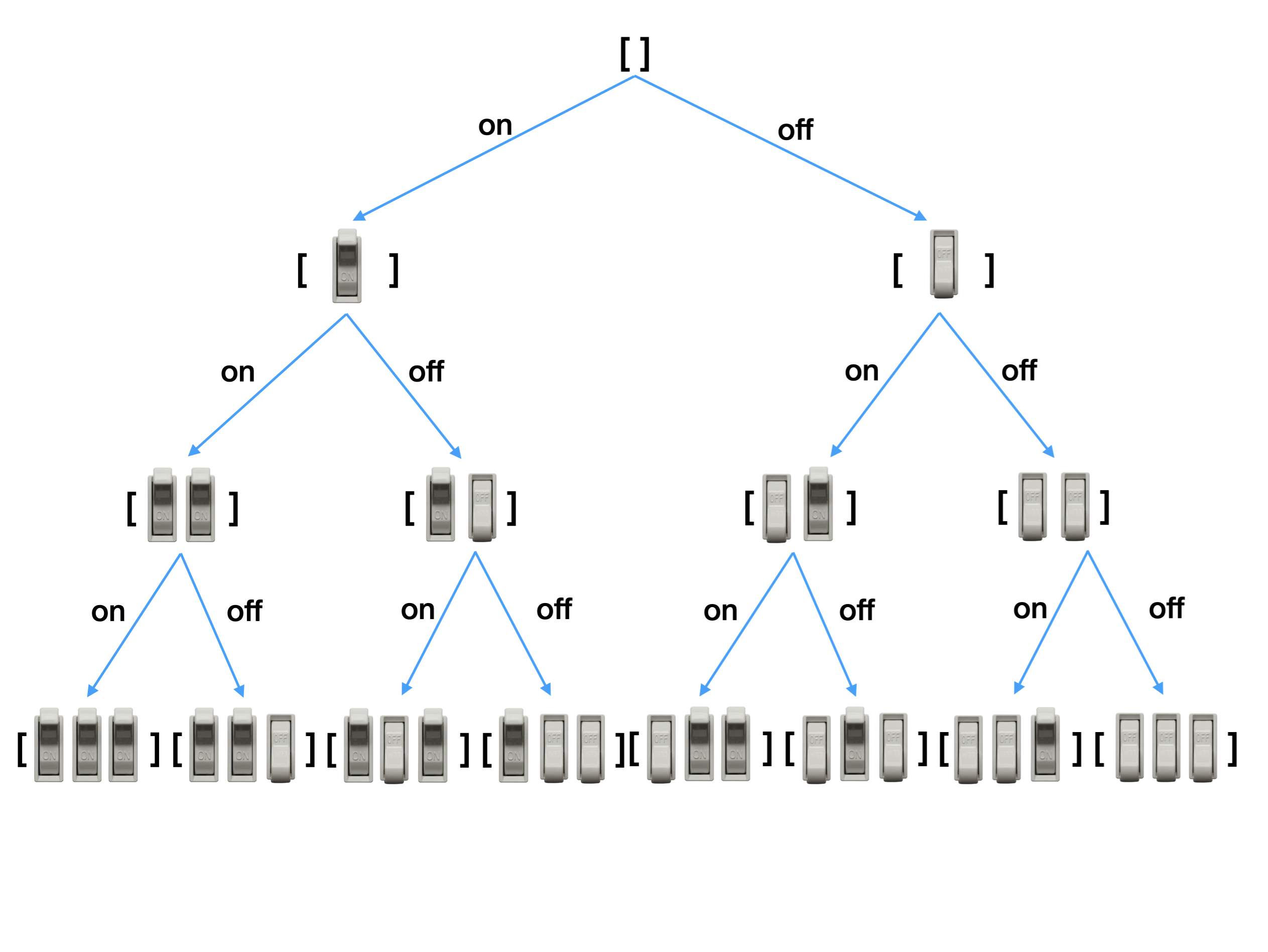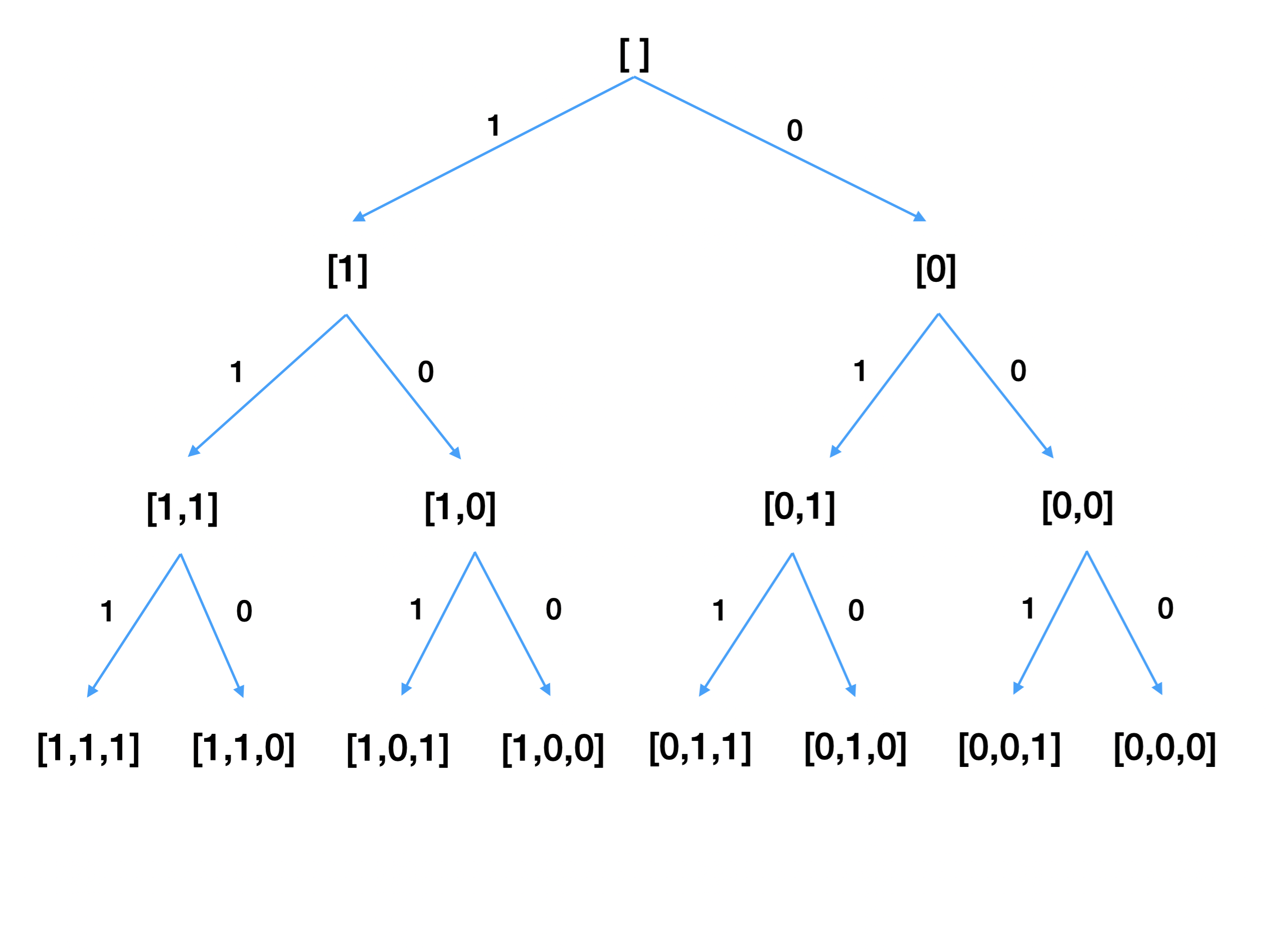# Math for Technical Interviews

If you prefer videos:

## How much math do I need to know for technical interviews?

Computer science is often associated with math, and some universities even place their computer science department under the math faculty. However, the reality is that you usually only need high school math for most of the software engineering interviews and day-to-day software engineering jobs.

#### But I have seen xxx question on LeetCode that needs yyy math trick

LeetCode has 2000+ questions, mainly user submitted. Having a particular question in the vast question bank doesn't mean very much. What really matters is whether the question is asked in an actual interview. If you look at the top questions companies ask, the questions that require advanced math tricks or knowledge are rarely asked. Questions that require knowing a particular math trick or fact are a knowledge test, and interviews are supposed to test your coding and problem-solving skills, not specific math knowledge.

#### What if I'm so unlucky that I got asked a tricky math question?

At most companies, candidates' performance ratings are reviewed by engineers other than the interviewers. And if a question is considered too difficult or off-topic, that round will be considered to carry less significance and assigned less weight in the final decision. So don't sweat about not knowing advanced math.

#### What if I don't even remember high school math?

You can learn it in one hour. Let's go through them now!

## Logarithmic

Logarithmic functions (log) are the inverses of exponential functions.

In computer science, log is base 2, because computers use binary numbers. Everything in a computer is store as 0's and 1's. This is different from other sciences, where 10 is the most common base.

Exponential:

`2^2 = 2 * 2 = 4`

`2^3 = 2 * 2 * 2 = 8`

`2^4 = 2 * 2 * 2 * 2 = 16`

The question `log(x)` answers is: how many `2` do we need to multiply to get `x`?

`log(8) = 3` we need to multiply three 2s, `2 * 2 * 2` to get `8`

`log(16) = 4` we need to multiply four 2s, `2 * 2 * 2 * 2` to get `16`

Another way to phrase it is: how many times can we divide `x` by `2` until we get to `1`? `8/2/2/2 = 1` we have to divide `8` by 2 three times to get to `1`, so `log(8) = 3`.

`16/2/2/2/2 = 1` we have to divide `16` by 2 four times to get to `1`, so `log(16) = 4`.

### Why is log important?

We sometimes want to reduce the problem size by half in computer algorithms. For example,

• in binary search, we cut the search range by (about) half each time. The time complexity is `log(n)`.
• in a full binary tree with `n` nodes, where each node except the leaves has two children, the tree's height is `log(n)`.
• and many other algorithms like merge sort, heap etc. We will look at them in the future sections.

## Permutations and factorial

A set is a group of things (called “elements”). There is no order between elements within a set. For example, `(a, b)`.

A permutation of a set is an arrangement of elements in the set into a sequence. The order does matter for permutations since a sequence has an order. Two sequences with the same elements but different ordering are considered different permutations. For example, a permutation of the set `(a, b)` is `[a, b]`, and the other is `[b, a]`.

The following figure shows all the permutations of `(a, b, c)`.### Counting permutations

To create a permutation, we have `3` choices for the first letter. The tree has three branches on the first level. After choosing our first letter, we have `2` letters left to choose from for our second letter. The tree has two branches on the second level. Finally, we have only `1` letter left for our third letter. The total number of permutations is `3 * 2 * 1 = 6`.

The number of permutations for a set of size `n` is `n * (n-1) * (n-2)... 1`. This is called factorial of `n`, denoted by `n!`. For example, `5! = 5 * 4 * 3 * 2 * 1 = 120`. This means there are `120` ways to arrange `5` letters in a row.

## Subsets

We call set B a subset of set A if every element in B is also found in A. For example, `(1, 3, 9)` is a subset of `(1, 2, 3, 5, 6, 7, 9)`.

### Counting subsets

How many subsets does a set have? Well, for each element, we can either choose to include it or not. Thus, for each element, there are 2 options. That means for `n` elements, we have `2 * 2 * ... * 2` (`n` times) options, equal to `2^n`.

Here's a figure to illustrate this. Imagine we have switches that can be `on` or `off`. If we have only one switch, then there can be `2^1 = 2` states. Two switches can have `2^2 = 4` states. Three switches can have `2^3 = 8` states.## Arithmetic sequence

An arithmetic sequence is a sequence of numbers such that the difference between the consecutive terms is constant. For example,

`1 2 3 4 5` is an arithmetic sequence because the difference between consecutive numbers is `1`.

`1 3 5 7 9` is an arithmetic sequence because the difference between consecutive numbers is `2`.

`1 2 4` is NOT an arithmetic sequence because `2-1=1` but `4-2=2`.

### Sum of an arithmetic sequence

The sum of an arithmetic sequence is `(first_element + last_element) * number_of_element / 2`. Here's the animated proof from Wikipedia if you are interested.

For example:

`sum([1,2,3,4,5]) = (1 + 5) * 5 / 2 = 15`

`sum([1,3,5,7,9]) = (1 + 9) * 5 / 2 = 25`

Because `last_element = first_element + difference * (number_of_element - 1)` , the sum can be expressed as `(2 * first_element + difference * (number_of_elements - 1)) * number_of_elements / 2`). In big O complexity analysis, we drop the constant terms (`first_element`, `diffence` and `-1`), so this really becomes `O(n^2)`.